## Saturday, June 25, 2016

### Understanding and Implementing Deepmind's DRAW Model

This post was first published on 2/27/16, and has since been migrated to Blogger.This blog post is a summary of Google Deepmind's paper DRAW: A Recurrent Neural Network For Image Generation. Also included is a tutorial on implementing it from scratch in TensorFlow.
The paper can seem a bit intimidating at first, but it is surprisingly intuitive once you look at it as a small (but elegant) extension to existing generative modeling techniques. Thanks to the TensorFlow framework, the implementation only takes 158 lines of Python code.

Below are some outputs produced by my DRAW implementation. The images on the left have the optional "attention mechanism" turned on, and the images on the right were generated with attention turned off.

DRAW w/ Attention DRAW w/o Attention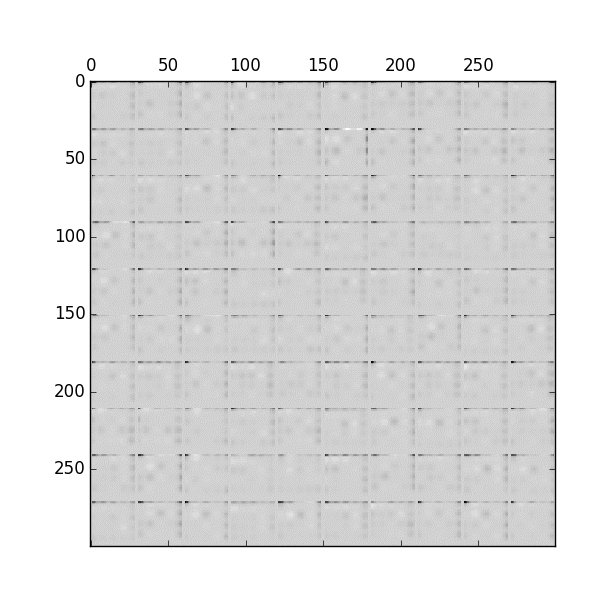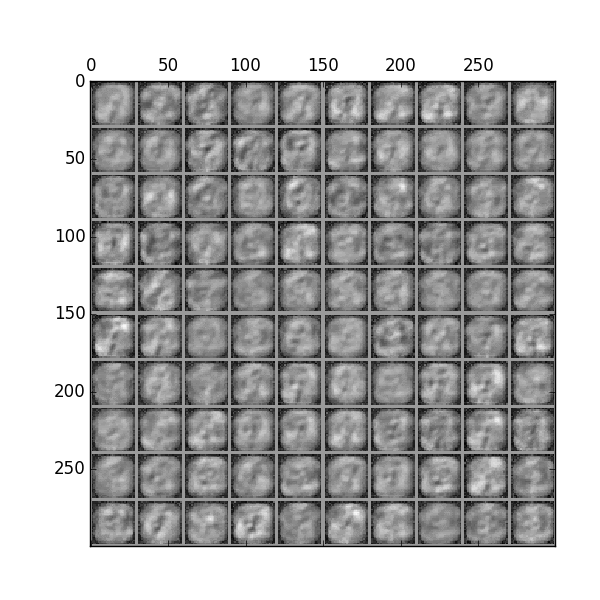It may not look like much, but the images are completely synthetic - we've trained a neural network to transform random noise into an endless stream of images that it has never even seen before. In a sense, it is "dreaming up" the images.

The full source code can be found on Github.

## A Story

Imagine that you crash land in the Sahara desert and meet a young boy who asks you to "draw him a sheep".

You draw a mutton chop in the sand with a stick.

"Now draw me a sample from the normal distribution," he says.

Sure - you bust open a Python shell on your laptop and type import numpy; numpy.random.normal()
"0.3976147272555", you tell him.

He asks you to draw another sample, so you run your code again and this time you get -0.27625249497.

"Now draw me a sample from the distribution of pictures where the camera operator forgot to take the cap off the lens"

You blink a couple times, then realize that there is only one such picture to choose from, so you draw him a black rectangle.

"Now draw me a sample from the distribution of pictures of houses."

"Erm... what?"

"Yes, houses! 400 pixels wide by 300 pixels tall, in RGB colorspace please. Don't put it in a box."

## Problem Statement

If you've ever written a computer program that used randomness, you probably learned how to sample the uniform probability distribution by calling rand() in your favorite programming language. If you were asked how to get a random number over the range $(0,2)$, or perhaps a random point in a unit square (2 dimensions) rather than a line (1 dimension), you could probably figure out how to do that too.

Now what if we were asked to "draw a sample from the distribution of valid covariance matrices?" We might actually care about this if we were trying to simulate an investment strategy on a portfolio of stocks. The equations get a lot harder, but it turns out we can still do it.

Now consider the distribution of house pictures (let's call it $P$) that the young boy asked us to sample from. $P$ is defined over a 400 pixel by 300 pixel space (120,000 pixels times 3 color channels = 360,000 numbers). Here are some samples drawn from that distribution via Reddit (these are real houses) .We'd like to be able to draw samples $c \sim P$, or basically generate new pictures of houses that may not even exist in real life. However, the distribution of pixel values over these kinds of images is very complex. Sampling the correct images needs to account for many long-range spatial and semantic correlations, such as:
• Adjacent pixels tend to be similar (a property of the distribution of natural images)
• The top half of the image is usually sky-colored
• The roof and body of the house probably have different colors
• Any windows are likely to be reflecting the color of the sky
• The house has to be structurally stable
• The house has to be made out of materials like brick, wood, and cement rather than cotton or meat
... and so on. We need to specify these relationships via equations and code, even if implicitly rather than analytically (writing down the 360,000-dimensional equations for $P$).
Trying to write down the equations that describe "house pictures" may seem insane, but in the field of Machine Learning, this is an area of intense research effort known as Generative Modeling.

## Generative Modeling Techniques

Formally, generative models allow us to create observation data out of thin air by sampling from the joint distribution over observation data and class labels. That is, when you sample from a generative distribution, you get back a tuple consisting of an image and a class label (e.g. "border collie"). This is in contrast to discriminative models, which can only sample from the distribution of class labels, conditioned on observations (you need to supply it with the image for it to tell you what it is). Generative models are much harder to create than discriminative models.

There have been some awesome papers in the last couple of years that have improved generative modeling performance by combining them with Deep Learning techniques. Many researchers believe that breakthroughs in generative models are key to solving unsupervised learning'', and maybe even general AI, so this stuff gets a lot of attention.
• Goodfellow et al. 2014 (with Yoshua Bengio's group in Montréal) came up with Generative Adversarial Networks (GAN), where a neural network is trained to directly learn the sampler function for the joint distribution. It is interactively pitted against another neural network that tries to reject samples from the generator while accepting samples from the "true" distribution .
• Gregor et al. 2014 (with Google Deepmind) proposed Deep AutoRegressive Networks (DARN). The idea is to use an autoencoder to learn some high-level features of some input, such as handwritten character images. The images can be reconstituted as a weighted combination of high-level features (e.g. individual pen strokes). To generate an entire image, we sample feature weights one at a time, conditioned on all the previous weights we have already chosen (ancestral sampling). We then let the decoding side of the autoencoder to recover the image from the feature vector .
• Kingma and Welling 2013 (Universiteit van Amsterdam) describe Variational AutoEncoders (VAE). Like the DARN architecture, we sample some feature representation (learned via autoencoder) generatively, then decode it into a meaningful observation. However, sampling this feature representation might be challenging if the feature interdependencies are complicated (or possibly cyclic). We use Variational methods to get around this: instead of trying to sample from the feature vector distribution $P$, we come up with a friendlier, tractable distribution $Q$ (such as a multivariate Gaussian) and instead sample from that. We vary the parameters $\theta$ of $Q$ so that $Q$'s shape is as similar to $P$ as possible.
• The combination of Deep Learning and Variational Bayes begs the question of whether we can also combine Deep Learning with VB's antithesis: MCMC methods. There are some groups doing work on this, but I'm not caught-up in this area. .

## DRAW: Core Ideas

Let's revisit the challenge of drawing houses.

The joint distribution we are interested in is $P(C)$. Joint distributions are typically written in terms of 2 or more variables, i.e. $P(X,Y)$, but distributions $P(C)$ over one multi-dimensional variable $C$ can also be thought of as joint distributions (think of $C$ as the concatenation of $X$ and $Y$).
The previous section discussed how neural networks can do all the hard work for us by either (1) learning the sampler function (GAN), (2) learning the directed Bayes net (DARN) or (3) learning the variational parameters (VAE). However, it's still asking a lot of our neural nets to figure out such a complex distribution. The feature space is massive ($\mathbb{R}^{360,000}$), and we might need tons of data to make this work.

This is where the DRAW paper comes in. The main contribution of the DRAW is that it extends Variational AutoEncoders with (1) "progressive refinement" and (2) "spatial attention". These two mechanisms greatly reduce the complexity burden that the autoencoder needs to learn, thereby allowing its generative capabilities handle larger, more complex distributions, like natural images.

### Core Idea 1: Progressive Refinement

Intuitively, it's easier to ask our neural network to merely "improve the image" rather than "finish the image in one shot". Human artists work by iterating on their canvas, and infer from their drawing what to fix and what to paint next.

If $P(C)$ is a joint distribution, we can rewrite it as $P(C)=P(A|B)P(B)$, where $P(A|B)$ is the conditional distribution and $P(B)$ is the prior distribution. We can think of $P(B)$ as another joint distribution, so $P(B)=P(B|D)P(D)$. So priors can have their own priors, which can have their own priors... it's turtles all the way down.

Now returning to the DRAW model, "progressive refinement" is simply breaking up our joint distribution $P(C)$ over and over again, resulting in a chain of latent variables $C_1, C_2, ... C_{T-1}$ to a new "observed variable distribution" $P(C_T)$, our house image.

$$P(C)=P(C_T|C_{T-1})P(C_{T-1}|C_{T-2})...P(C_1|C_0)P(0)$$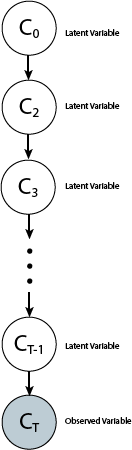The trick is to sample from the "iterative refinement" distribution $P(C_t|C_{t-1})$ several times rather than straight-up sampling from $P(C)$. To reuse the house images example, sampling $P(C_1|C_0)$ might be only responsible for getting the sky right, sampling $P(C_2|C_1)$ might be only responsible for capturing proper windows and doors (after we've gotten the sky right), and so on.
In the case of the DRAW model, $P(C_t|C_{t-1})$ is the same distribution for all $t$, so we can compactly represent this as the following recurrence relation (if not, then we have a Markov Chain instead of a recurrent network) .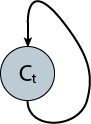### Core Idea 2: Spatial Attention

Progressive refinement simplifies things by splitting up the generative process over a temporal domain. Can we do something similar in a spatial domain?

Yes! In addition to only asking the neural network to improve the image a little bit on each step, we can further reduce the compexity burden by asking it to only improve a small region of the image at a time. The neural network can devote its "mental resources" to one local patch rather than having to think think about the distribution of improvement across the entire canvas. Because "drawings over small regions" are easier to generate than "drawings over large regions", we should expect things to get easier for the network.

Easier:Harder: the above face is in there somewhere! Artwork by Kim Jung gi.Of course, there are some subtleties here: how big should the attention patch be? Should the "pen-strokes" be sharp or blurry? How do we get the attention mechanism to be able to support both wide, broad strokes and little precise refinements?

Fear not. As we will see later, these are dynamic parameters that will be learned by the DRAW model.

## The Model

The DRAW model uses recurrent networks (RNNs) that run for $T$ steps of progressive refinement. Here is the neural network, where the RNNs have been unrolled across time - everything is feedforward now.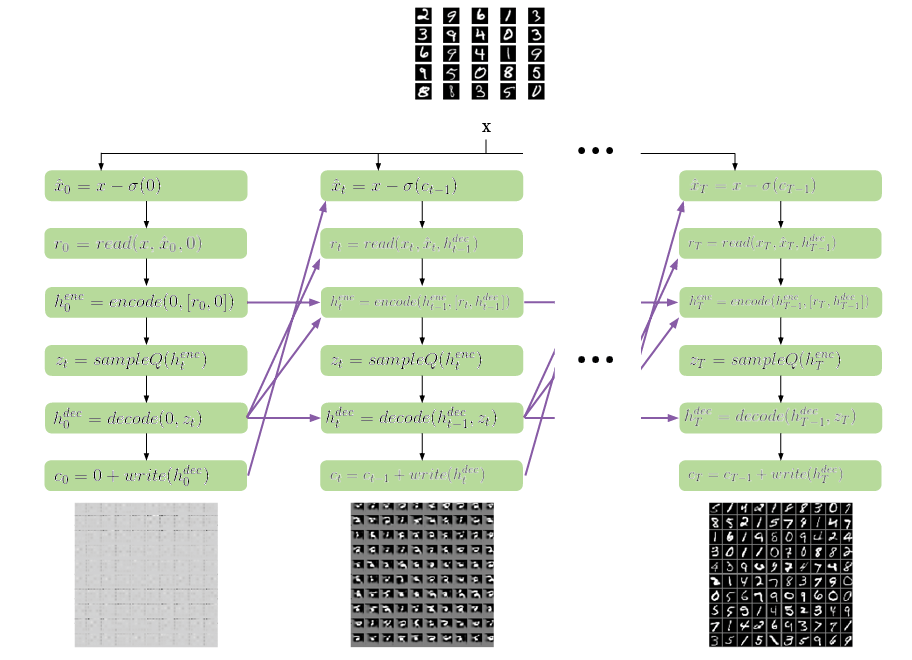To keep things concrete, here are the shapes of the tensors being passed along the network: A and B are the image width and height respectively (so the image is a B x A matrix), and N is the width of the attention window (in pixels). It is standard practice to flatten 2D image data entirely into the second dimension (index=1) because this construction generalizes better when we want to use our neural network on other data formats.

Tensor Shape
$x$ (batch_size, B*A)
$\hat{x}$ (batch_size,B*A)
$r_t$ (batch_size,2*N*N)
$h_t^{enc}$ (batch_size,enc_size)
$z_t$ (batch_size,z_size)
$h_t^{dec}$ (batch_size,dec_size)
$write(h_t^{dec})$ (batch_size,B*A)

$h_t^{enc}$, $z_t$, and $h_t^{dec}$ form the "Variational Autoencoder" component of the model. Images seen by the network are "compressed" into features by the encoding layer. The reason why we want to use an autoencoder is because it's too difficult to sample images in pixel space directly. We want to find a more compact representation for the images we are trying to generate, and instead sample from those representations before transforming them back into images.

To sample $h^{enc}$ generatively, we pray to the Variational Bayes gods and hope that there is some $(\mu,\Sigma)$ that parameterizes a z_size-dimensional multivariate Gaussian $Q$ in a way such that $Q$ looks kinda like $P(h_{enc})$. We choose Gaussian because it's well-studied and easy to sample from, but you could have also chosen a multivariate Gamma, Uniform, or other distributions as long as you can match it to $P(h^{enc})$.

Expressing this model is easy in TensorFlow. The syntax is similar to manipulating Numpy arrays, so we practically can copy equations right out of the paper: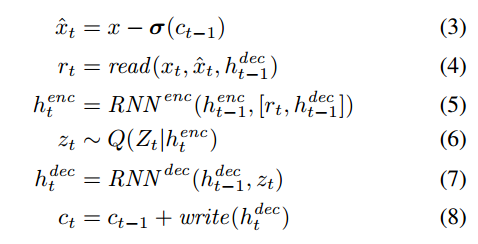### Note about Weight Sharing

Because the model is recurrent, we want to be using the same network weights for all our linear layers and LSTM layers at each time step. tf.variable_scope(reuse=True) is used to share the parameters of the network.

Annoyingly though, we have to manually disable reuse=True on the first time step, otherwise trying to create a new variable with tf.get_variable(reuse=True) raises an "under-share" exception. That's what the global variable DO_SHARE is for: weight sharing is turned off for t=0, then we switch it on permanently.

### Reading

The implementation of read without attention is super easy: simply concatenate the image with the error image (difference between the image and the current reconstruction. I highly recommend implementing the non-attentive version of DRAW first, in order to check that all the other components work.

To implement read-with-attention, we use a basic linear layer to compute the 5 scalar parameters that we use to set up the read filterbanks $F_x$ and $F_y$. I made a short helper function linear to help with other places in the model where affine transformations $W$ are needed.

You might be wondering - why do we compute $log(\sigma)$, $\log(\gamma)$, etc. rather than just have the linear layer spit out $\sigma$ and $\gamma$?. I think this is so that we guarantee $\sigma=exp(log(\sigma))$ and $\gamma=exp(log(\gamma))$ are positive. We could just use tf.min and clamp the values, but it doesn't differentiate as well so our error gradients would propagate slower if we did that.

Once we obtain the filterbank parameters, we need to actually set up the filters. $F_X$ and $F_Y$ each specify $N$ 1-dimensional Gaussian bumps. These bumps are identical except that they are translated (mean-shifted) from each other at intervals of $\delta$ pixels, and the whole grid is centered at $(g_Y,g_X)$. Spacing these filters out means that we are downsampling the image, rather than just zooming really close.

The extracted value for attention grid pixel $(i,j)$ is the convolution of the image with a 2D Gaussian bump whose $x$ and $y$ components are the respective $i$ and $j$ rows in $F_X$ and $F_Y$. Thus, reading with attention extracts a N*N patch from the image patch and N*N from the error patch).

If you didn't follow what the last couple paragraphs said, that's okay: it's much easier to explain what the filterbanks are doing with a picture: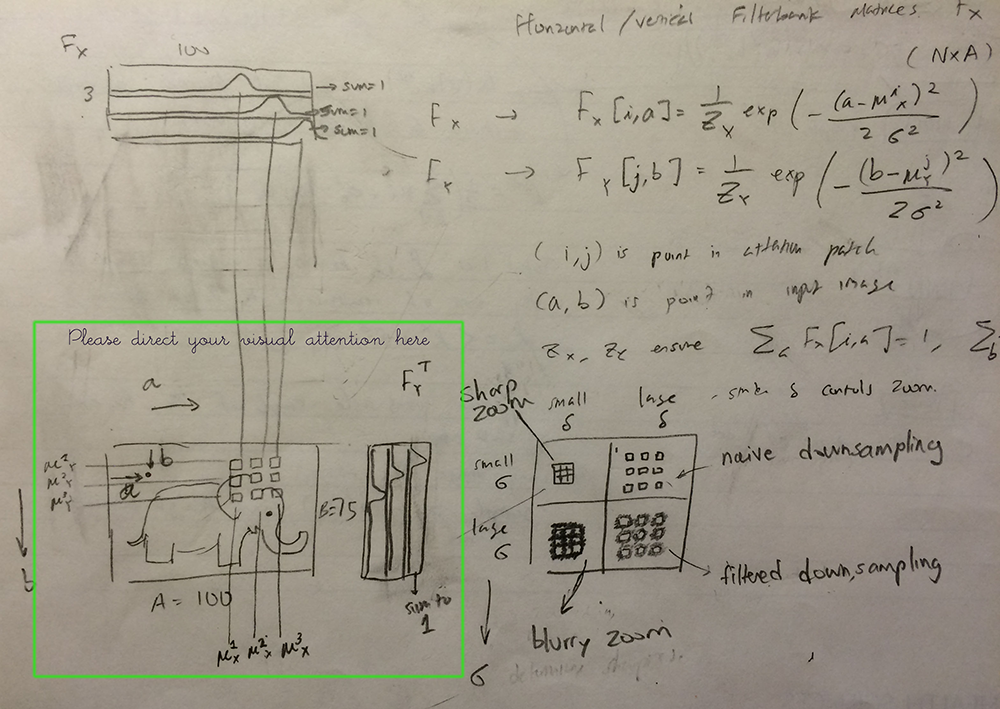Here's the code to build up the filterbank matrices $F_X$, $F_Y$.

### Batch Multiply vs. Vectorized Multiplication

In batched operations, we usually have tensors with the shape (batch_size,img_size). But the recipe calls for $F_YxF_X^T$, which is a matrix-matrix-matrix multiplication. How do we perform the multiplication when our $x$ is a vector?

As it turns out, TensorFlow provides a convenient tf.batch_matmul() function that lets us do exactly this. We temporarily reshape our $x$ vectors into (batch_size,B,A), apply batch multiplication, then reshape it back to a 2D matrix again.

Here is the read function with attention, using batch multiplication:

There's also a way to do this without tf.batch_matmul, and that is to just vectorize the multiplications. It's slightly verbose, but useful. Here is how you do it:

### Encoder

In TensorFlow, you build recurrent nets by specifying the time-unrolled graph manually (as drawn in the diagram above).

The constructor tf.models.rnn.rnn_cell.LSTMCell(output_size, input_size) returns to you a function, lstm_op corresponding to the update equations for an LSTM layer. You pass in the current state and input to lstm_op, and it returns the output and a new LSTM state (for which you are responsible for passing back into the next call to lstm_op).
output, new_state = lstm_op(input, current_state)

### Sampler

Given one instance of an encoded representation $h_t^{enc}$ of a "6" image, we'd like to map that to the distribution $P$ of all "6" images, or at least a variational approximation $Q \sim P$ of it. In principle, if we choose the correct $(\mu$, $\Sigma)$ to characterize $Q$, then we can sample other instances of "encoded 6 images". Note that the samples would all have random variations, but nonetheless still be images of "6".

If we save a copy of $(\mu,\Sigma)$, then we can create as many samples from $Q$ as we want using numpy.random.multivariate_normal(). In fact, the way to use DRAW as a generative model is to cut out the first half of the model (read, encode, and sampleQ), and just pass our own $z \sim \mathcal{N}(\mu,\Sigma)$ straight to the second half of the network. The decoder and writer transforms the random normals back into an image.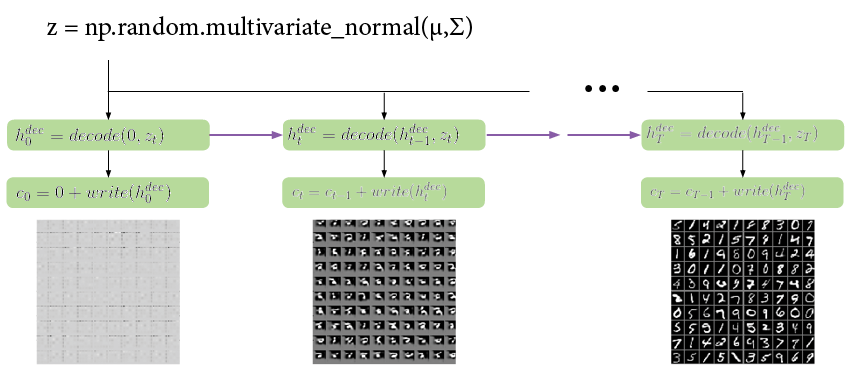Radical.

### Sampler Reparameterization Trick

There's one problem: if we're training the DRAW architecture end-to-end via gradient descent, how the heck do we backpropagate loss gradients through a function like numpy.random.multivariate_normal()?

The answer proposed in Kingma's Variational Autoencoders paper is hilariously simple: just rewrite $z=\mathcal{N}(\mu,\sigma)$ as $z=\mu+\mathcal{N}(0,1)*\sigma$. Now we can sort of take derivatives: $\partial{z}/\partial{\mu}=1, \partial{z}/\partial{\sigma}=e$ where $e \sim N(0,1)$.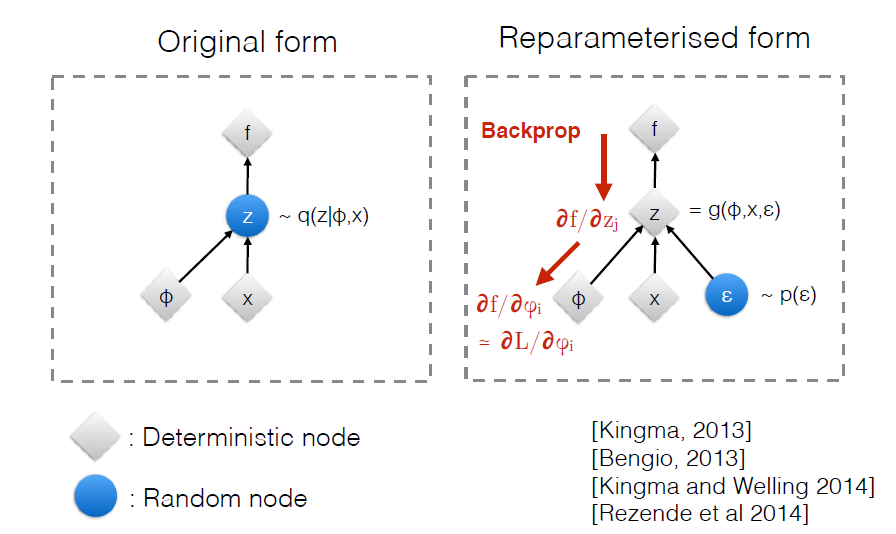In Karol Gregor's talk , $e$ is described as "added noise" that limits the information passed between the encoder and decoder layers (otherwise $\mu,\Sigma$, being made up of real numbers, would carry an infinite number of bits). Somehow this prevents similar $z$ being decoded into wildly different images, but I don't fully understand the info-theory interpretation quite yet.

### Decoder

The variable names in the paper make it a little confusing as to what exactly is being encoded and decoded at each layer. It's clear that $x_t, r_t, c_t$ are are in "image space", and $h^{enc}_t$ refers to the encoded feature representation of an image.

However, my understanding is that $h^{dec}_t$ is NOT the decoded image representation, but rather it resides in the same feature space as $h^{enc}_t$. The superscript "dec" is misleading because it actually refers to the "decoding of $z$ back into encoded image space". I think this is so because passing data through sampleQ is implicitly adding an additional layer of encoding.

It then follows that the actual transformation from "encoded image space" back to "image space" is done implicitly by the write layer. I'd love to hear an expert opinion on this matter though .

### Writing with Attention

During writing, we apply the opposite filter transformation we had during reading. Instead of focusing a large image down to a small "read patch", we now transform a small "write patch" to a larger region spanning the entire canvas. If we make the write patch pure white, we can visualize where the write filterbanks cause the model to attend to in the image. This can be useful for debugging or visualizing the DRAW model in action. To visualize the same thing with read filterbanks, just apply them as if they were write filters.

### Model Variables

It's usually a good idea to double-check the parameters you're optimizing over, and that variables are being properly shared, before switching training on. Here are the variables (learnable parameters) of the DRAW model and their respective tensor shapes.

  read/w:0 : TensorShape([Dimension(256), Dimension(5)])
read/b:0 : TensorShape([Dimension(5)])
encoder/LSTMCell/W_0:0 : TensorShape([Dimension(562), Dimension(1024)])
encoder/LSTMCell/B:0 : TensorShape([Dimension(1024)])
mu/w:0 : TensorShape([Dimension(256), Dimension(10)])
mu/b:0 : TensorShape([Dimension(10)])
sigma/w:0 : TensorShape([Dimension(256), Dimension(10)])
sigma/b:0 : TensorShape([Dimension(10)])
decoder/LSTMCell/W_0:0 : TensorShape([Dimension(266), Dimension(1024)])
decoder/LSTMCell/B:0 : TensorShape([Dimension(1024)])
writeW/w:0 : TensorShape([Dimension(256), Dimension(25)])
writeW/b:0 : TensorShape([Dimension(25)])
write/w:0 : TensorShape([Dimension(256), Dimension(5)])
write/b:0 : TensorShape([Dimension(5)])

Looks good!

### Loss Function

The DRAW model's loss function is $L_x+L_z$, or the sum of a reconstruction loss (how good the picture looks) and a latent loss for our choice of $z$ (a measure of how bad our variational approximation is of the true latent distribution). Because we are using binary cross entropy for $L_x$, we need our MNIST image values to go from 0-1 (binarized) rather than -0.5 to 0.5 (mean-normalized). The latent loss is the the standard reverse-KL diverence used in variational methods.
I don't have a full understanding of this yet, so maybe I'll ask here: why are we allowed to just combine reverse-KL with binary cross entropy? If we only minimize $L_x$, it looks like we can still obtain good drawings even though the latent loss increases. This suggests that KL and binary cross entropy are apples and oranges, and we can change the cost function to $Lx+\lambda*Ly$ where $\lambda$ allows us to prioritize one kind of loss over another (or even alternate optimizing $L_x$ and $L_z$ in an EM-like fashion).

One very annoying bug that I encountered was that while DRAW without attention trained without problems, adding attention suddenly caused numerical underflow problems to occur during training. The training would start off just fine, but soon all of the gradients would explode into NaNs.
The problem was that I was passing near-zero values to the log function used in binary cross entropy, even though $x_{recons}=\sigma(c_T)$ has an asymptote at 0. The fix was to just add a small positive epsilon-value to what I was passing into log, to really make sure it was strictly positive.

### Optimization

The code for setting up an Adam Optimizer to minimize the loss function is not super interesting to look at, so I leave you to take a look at the full code on Github. It's common in Deep Learning to apply momentum to the optimizer and clip gradients that are too large, so I utilized those heuristics in my implementation (but they are not necesary). Here is a plot of the training error for DRAW with attention: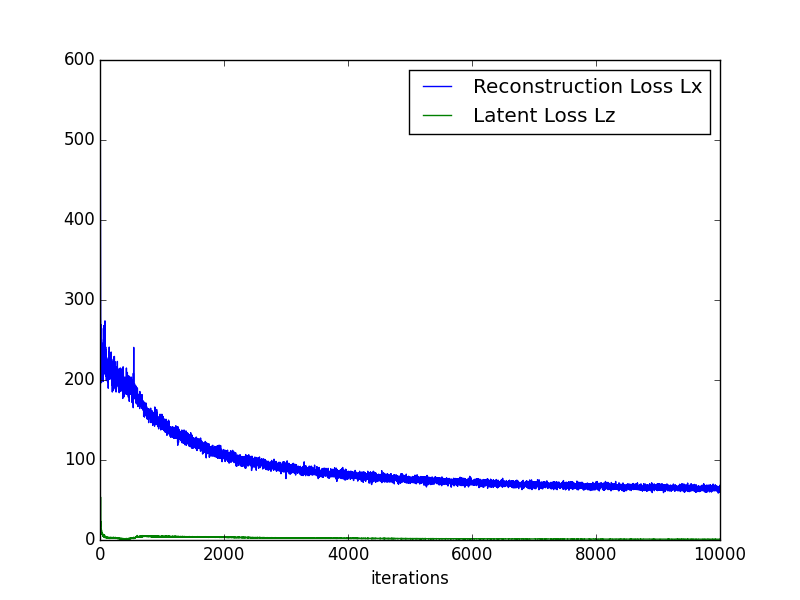Here's an example of MNIST being generated after training. Notice how the attention mechanism "traces" out the curves of the letter, rather than "blotting" it in gradually (DRAW without attention).## Closing Thoughts

DRAW improves generative modeling capabilities of Variational AutoEncoders by "splitting" the complexity of the task across the temporal and spatial domains (progressive refinement and attention). TensorFlow made this a joy to implement, and I'm excited what other exotic differentiable programs will come out of the Deep Learning woodwork. Here are some miscellaneous thoughts:
• It's quite remarkable that even though this model perceives and manipulates images, there was no use of convolutional neural nets. It's almost as if RNNs equipped with attention can somehow do spatial tasks that much-larger convnets are so good at. I'm reminded of how Baidu actually uses convnets in their speech recognition models (convolution across time rather than space), rather than LSTMs (as Google does). Is there some sort of representational duality between recurrent and convolutional architectures?
• Although the sampleQ block in the DRAW model is implemented using a Variational AutoEncoder, theoretically we could replace that module with one of the other generative modeling techniques (GAN, DARN), so long as we are still able to backpropagate loss gradients through it.
Please don't hesitate to reach out to me via email if there are factual inaccuracies/typos in this blog post, or something that I can clarify better. Thank you for reading!

## Footnotes

1. My previous blog post explains Generative Adversarial Networks in more detail (it uses sorting and stratified sampling as I had not learned about the reparameterization trick at the time).
2. The prefix "auto" comes from the use of an autoencoder and the suffix "regressive" comes from the correlation matrix of high-level features used in the conditional distribution. Karol Gregor's tech talk on DARN explains the idea very well.
3. Introduction to MCMC for Deep Learning and Learning Deep Generatie Models with Doubly Stochastic MCMC
4. I highly recommend checking out the "HousePorn" subreddit (SFW) https://www.reddit.com/r/Houseporn/
5. "Progressive refinement" is why recurrent neural networks generally have far greater expressive power than feedforward networks of the same size: each step of the RNN's state activation is only responsible for learning the "progressive refinement" distribution (or next word in a full sentence), whereas a model that isn't recurrent has to figure an entire sequence out in one shot. Cool!
6. Karol Gregor's guest lecture in Oxford's Machine Learning class taught by Nando de Freitas. I highly recommend this lecture series, as it contains more advanced Deep Learning techniques (heavily influenced by Deepmind's research areas).
7. This is a somewhat simplistic reduction: I think that the representation that a layer ends up learning probably has a lot to do with how much size it is allocated. There's no reason why the feature representation $h^{dec}_t$ learns would resemble that of $h^{enc}_t$ if their layer sizes are different.

#### 8 comments:

1.This comment has been removed by the author.

2.Hi, Eric.
Thanks a lot for a great and very helpful post !
Going through the code, I might have some misunderstanding in the implementation of Lz (or there might be a problem ?)
I would expect that line 16 in draw_loss.py above would be:
kl_terms[t]=0.5*tf.reduce_sum(mu2+sigma2-2*logsigma,1)-z_size*.5

instead of:
kl_terms[t]=0.5*tf.reduce_sum(mu2+sigma2-2*logsigma,1)-T*.5

Am I missing something ?

Again, Great work ! thanks a lot !!
Guy

3.Thanks for writing this - excellent post.

4.Happy for getting this good content about Deepmind's DRAW Mode.Thanks a lot for sharing this impementation details of this kind of model.Happy to read this and getting lots of information from your post.Are you need essay writing services helps?Its very useful for your academic works and the available writing services like best essay writing service,custom essays and so on are very useful and helpful services,students can gain custom essays to overcome this weakness and move towards achieving a sustainable and successful career.

5.Thank you Eric, this really helped me solidify my understanding of the paper.

6.Great scott!
The paper felt like I was reading latin.
This is so much easier to understand. Thank you!!

7.It is awesome. Now I realize that, how tough that this profession is. Great job done so far. Thanks for all thing you have done. Custom Essay Writing Services

8.Great work. Thanks for this article. Do my Essays is one article which you may be searching for. Read it.

Comments will be reviewed by administrator (to filter for spam and irrelevant content).